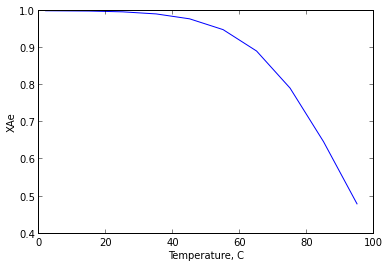Chapter 9 : Temperature and Pressure Effects¶

Example 9.1 page no : 209¶

In :
import math

# Variables
CpA = 35.            # j/mol.K
CpB = 45.             # j/mol.K
CpR = 70.            # j/mol.K
T1 = 25.             # C
T2 = 1025.           # C
Hr = -50000.

# Calculations
#Enthalpy balance for 1mol A,1 mol B,2 mol R
nA = 1.
nB = 1.
nR = 2.
dH = nA*CpA*(T1-T2)+nB*CpB*(T1-T2)+(Hr)+nR*CpR*(T2-T1);

# Results
print " dHJ at temperature 1025C is %.f "%(dH)
if dH>0 :
print "Reaction is Exothermic"
else:
print "Reaction is endothermic at 1025OC"
dHJ at temperature 1025C is 10000
Reaction is Exothermic

Example 9.2 page no : 213¶

In :
%matplotlib inline

import math
from matplotlib.pyplot import *
from numpy import *

Ho = -75300.                       # J/mol
Go = -14130.                       # J/mol
R = 8.3214
T1 = 298.

# Calculations
#With all specific heais alike,dCp = 0
Hr = -Ho;
K298 = math.exp(-Go/(R*T1));

#Taking different values of T
T1 = array([2,15,25,35,45,55,65,75,85,95])                  #degree celcius
T = array([278,288,298,308,318,328,338,348,358,368])        #kelvin

XAe = zeros(10)

for i in range(10):
K = K298*math.exp((Hr/R)*((1./T[i])-(1./298)));
XAe[i] = K/(K+1);

# Results
plot(T1,XAe)
xlabel("Temperature, C")
ylabel("XAe")
print (" From the graph we see temp must stay below 78 C if conversion of 75% or above is expected")
show()
Populating the interactive namespace from numpy and matplotlib
From the graph we see temp must stay below 78 C if conversion of 75% or above is expected
WARNING: pylab import has clobbered these variables: ['draw_if_interactive']
%pylab --no-import-all prevents importing * from pylab and numpyExample 9.3 page no : 217¶

In :
import math

# Variables

# from example 9.2
XA = 0.581;
t = 1.                    #min
XAe = 0.89;
XAe1 = 0.993;
T1 = 338.
T2 = 298.
R = 8.314

# Calculations
k1_338 = -(XAe/t)*math.log(1-(XA/XAe));
XA1 = 0.6;
t1 = 10.                #min

k1_298 = -(XAe1/t1)*math.log(1-(XA1/XAe1));
E1 = (R*math.log(k1_338/k1_298))*(T1*T2)/(T1-T2)
ko = k1_338/(math.exp(-E1/(R*T1)))

# Results
print " The rate constants are k = exp[75300/RT-24.7] min-1"
print " k1 = exp[17.2-48900/RT] min-1"
print " k2 = exp[41.9-123800/RT] min-1 "
print " E1 = %.2f"%E1
print " K0 = %.2f"%ko
The rate constants are k = exp[75300/RT-24.7] min-1
k1 = exp[17.2-48900/RT] min-1
k2 = exp[41.9-123800/RT] min-1
E1 = 48679.81
K0 = 31411847.30

Example 9.4 page no : 229¶

In :
# Variables
CAo = 4.                    #mol/litre
FAo = 1000.                 #mol/min
A = 0.405                   #litre/mol.min

# Calculations
t = CAo*A;
V = FAo*A;

# Results
print " Part a"
print " The space time needed is %.2f min"%(t)
print " The Volume needed is %.f litres"%(V)

# Note : We do not have value of 'rA'. so part B can not be calculated and plotted
Part a
The space time needed is 1.62 min
The Volume needed is 405 litres

Example 9.5 pageno : 231¶

In :
# Variables
CAo = 4.                # mol/liter
FAo = 1000.             # mol/min
XA = 0.8;               # %
Cp = 250.               #cal/molA.K
Hr = 18000.             #cal/molA
rA = 0.4;

# Calculations and Results
V = FAo*XA/rA;

print " Part a"
print " The size of reactorlitres) needed is %.f litres"%(V)

slope = Cp/Hr;
#Using graph
Qab1 = Cp*20;               #cal/molA
Qab = Qab1*1000;            #cal/min
Qab = Qab*0.000070;         #KW

print " Part b"
print " Heat Duty of precooler is %.2f kW"%(Qab)

Qce1 = Cp*37;               #cal/molA fed
Qce = Qce1*1000;            #cal/min
Qce = Qce*0.000070;         #KW

print " Heat Duty of postcooler is %.2f kW"%(Qce)

# answers may vary because of rounding error.
Part a
The size of reactorlitres) needed is 2000 litres
Part b
Heat Duty of precooler is 350.00 kW
Heat Duty of postcooler is 647.50 kW

Example 9.6 pageno : 233¶

In :
# Variables
FAo = 1000.         #mol/min
Area = 1.72;

# Calculations
V = FAo*Area;

# Results
print " The volume of adiabatic plug flow reactor is %.f litres"%(V),
The volume of adiabatic plug flow reactor is 1720 litres

Example 9.7 page no : 234¶

In :
# Variables
FAo = 1000.             #mol/min
Area = (0.8-0)*1.5;

# Calculations
V = FAo*Area;

# Results
print " The volume required is %.f litres"%(V),
The volume required is 1200 litres
In [ ]: